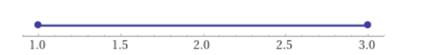# The solution of the inequality 4 x − 3 ≥ x 2 graphically.### Precalculus: Mathematics for Calcu...

6th Edition
Stewart + 5 others
Publisher: Cengage Learning
ISBN: 9780840068071### Precalculus: Mathematics for Calcu...

6th Edition
Stewart + 5 others
Publisher: Cengage Learning
ISBN: 9780840068071

#### Solutions

Chapter 1, Problem 97RE
To determine

## To calculate: The solution of the inequality 4x−3≥x2 graphically.

Expert Solution

[1,3]

### Explanation of Solution

Given information:

The inequality is 4x3x2

Calculation:

Consider the inequality 4x3x2 .

Subtract both sides by x2 .

4x3x24x3x2x2x24x3x20(x3)(x1)0x1,x3

So, the solution is [1,3] .

The solution can be graphed on number line as shown below.### Have a homework question?

Subscribe to bartleby learn! Ask subject matter experts 30 homework questions each month. Plus, you’ll have access to millions of step-by-step textbook answers!